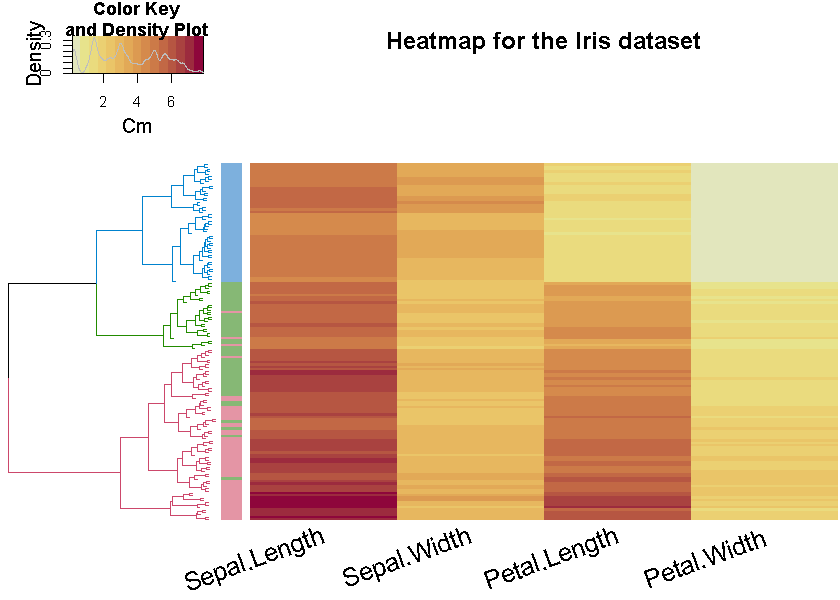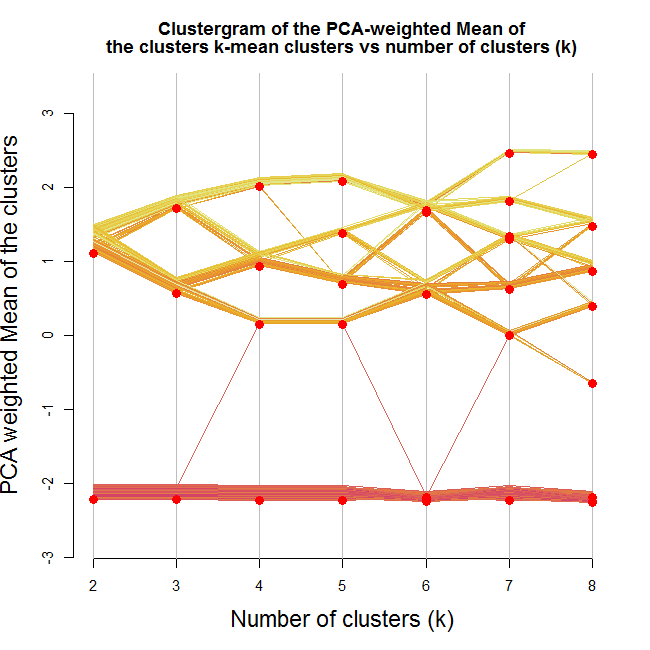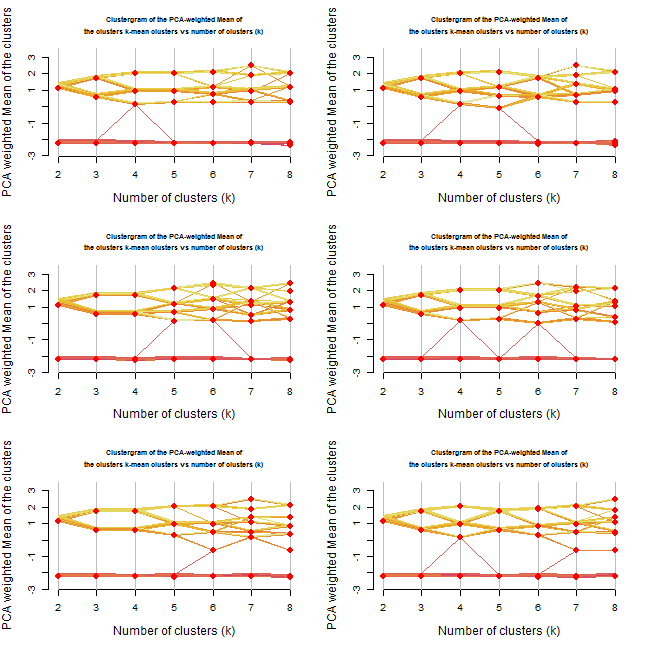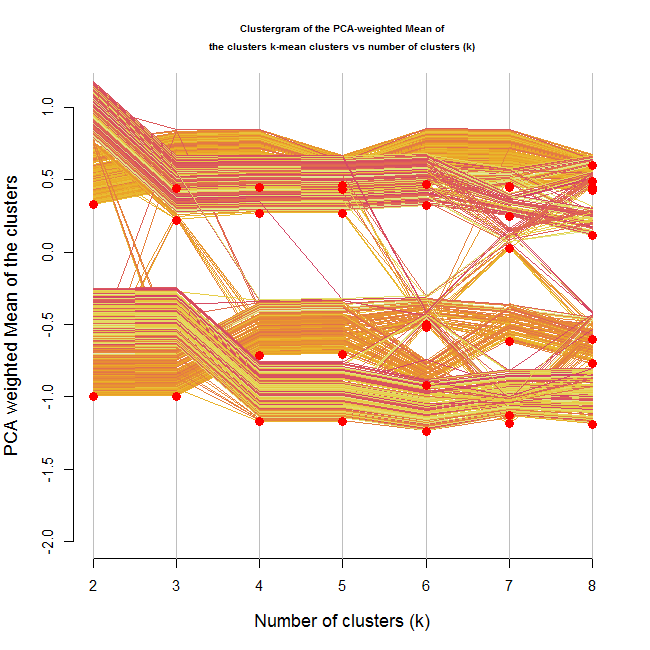## heatmaply: interactive heat maps (with R)

I am pleased to announce heatmaply, my new R package for generating interactive heat maps, based on the plotly R package.

### tl;dr

By running the following 3 lines of code:

```install.packages("heatmaply")
library(heatmaply)
heatmaply(mtcars, k_col = 2, k_row = 3) %>% layout(margin = list(l = 130, b = 40))
```

You will get this output in your browser (or RStudio console):

## Slides from my JSM 2015 talk on dendextend

If you happen to be at the JSM 2015 conference this week, then this Monday, at 2pm, I will give a talk on the dendextend R package  (in the session “Advances in Graphical Frameworks and Methods Part 1“) – feel free to drop by and say hi.

Here are my slides for the intended talk:

p.s.: Yes – this presentation is very similar, although not identical, to the one I gave at useR2015. For example, I mention the new bioinformatics paper on dendextend.

## dendextend: a package for visualizing, adjusting, and comparing dendrograms (based on a paper from “bioinformatics”)

This post on the dendextend package is based on my recent paper from the journal bioinformatics (a link to a stable DOI). The paper was published just last week, and since it is released as CC-BY, I am permitted (and delighted) to republish it here in full:

## abstract

Summary: dendextend is an R package for creating and comparing visually appealing tree diagrams. dendextend provides utility functions for manipulating dendrogram objects (their color, shape, and content) as well as several advanced methods for comparing trees to one another (both statistically and visually). As such, dendextend offers a flexible framework for enhancing R’s rich ecosystem of packages for performing hierarchical clustering of items.

Availability: The dendextend R package (including detailed introductory vignettes) is available under the GPL-2 Open Source license and is freely available to download from CRAN at: (https://cran.r-project.org/package=dendextend)

Contact: [email protected]

## dendextend version 1.0.1 + useR!2015 presentation

When using the dendextend package in your work, please cite it using:

Tal Galili (2015). dendextend: an R package for visualizing, adjusting, and comparing trees of hierarchical clustering. Bioinformatics. doi:10.1093/bioinformatics/btv428

My R package dendextend (version 1.0.1) is now on CRAN!

The dendextend package Offers a set of functions for extending dendrogram objects in R, letting you visualize and compare trees of hierarchical clusterings. With it you can (1) Adjust a tree’s graphical parameters – the color, size, type, etc of its branches, nodes and labels. (2) Visually and statistically compare different dendrograms to one another.

The previous release of dendextend (0.18.3) was half a year ago, and this version includes many new features and functions.

To help you discover how dendextend can solve your dendrogram/hierarchical-clustering issues, you may consult one of the following vignettes:

Here is an example figure from the first vignette (analyzing the Iris dataset)This week, at useR!2015, I will give a talk on the package. This will offer a quick example, and a step-by-step example of some of the most basic/useful functions of the package. Here are the slides:

Lastly, I would like to mention the new d3heatmap package for interactive heat maps. This package is by Joe Cheng from Rstudio, and integrates well with dendrograms in general and dendextend in particular (thanks to some lovely github-commit-discussion between Joe and I). You are invited to see lively examples of the package in the post at the RStudio blog. Here is just one quick example:

d3heatmap(nba_players, colors = “Blues”, scale = “col”, dendrogram = “row”, k_row = 3)## The dendextend package for visualizing and comparing trees of hierarchical clusterings (slides from useR!2014)

When using the dendextend package in your work, please cite it using:

Tal Galili (2015). dendextend: an R package for visualizing, adjusting, and comparing trees of hierarchical clustering. Bioinformatics. doi:10.1093/bioinformatics/btv428

This week I presented in the useR!2014 my package dendextend (also on github), for easily manipulating, visualizing, and comparing dendrograms. Put simply, it is a package designed to easily create figures like these:Here is my presentation from useR:

[gview file=”https://www.r-statistics.com/wp-content/uploads/2014/07/2014-07-01-useR2014-03.pdf” profile=”null” save=”1″]

You are also invited to give a look to the current version of the package vignettes:

https://github.com/talgalili/dendextend/blob/master/vignettes/dendextend-tutorial.pdf

I highly welcome features suggestions and bug reports (or just “wow, this is awesome”) sent to my e-mail (tal.galili AT gmail.com), you can also leave a comment or use the github issue page.

A sidenote on useR!2014: this year’s useR conference was wonderful! I enjoyed the many talks, sessions, posters, and especially the so many wonderful R users I got to meet (and I will not try to list all of you – but you know who you are, and how much I enjoyed seeing you!). As corny as it may sound – we, the people who use R, are truly a community. There is a lot to be said about getting to meet so many people who share my own passion for statistical programming, open source, collaboration, open science, and a better future in general. Gladly, you can get a sense of what happened there by having a look at the twitter hashtag #useR2014. Several great R bloggers already started writing about it, you can see their posts here: 1, 2, 3, 4, 5. And I hope more posts will follow. I hope to see you in next year’s useR!2015!

## Clustergram: visualization and diagnostics for cluster analysis (R code)

### About Clustergrams

In 2002, Matthias Schonlau published in “The Stata Journal” an article named “The Clustergram: A graph for visualizing hierarchical and . As explained in the abstract:

In hierarchical cluster analysis dendrogram graphs are used to visualize how clusters are formed. I propose an alternative graph named “clustergram” to examine how cluster members are assigned to clusters as the number of clusters increases.
This graph is useful in exploratory analysis for non-hierarchical clustering algorithms like k-means and for hierarchical cluster algorithms when the number of observations is large enough to make dendrograms impractical.

A similar article was later written and was (maybe) published in “computational statistics”.

Both articles gives some nice background to known methods like k-means and methods for hierarchical clustering, and then goes on to present examples of using these methods (with the Clustergarm) to analyse some datasets.

Personally, I understand the clustergram to be a type of parallel coordinates plot where each observation is given a vector. The vector contains the observation’s location according to how many clusters the dataset was split into. The scale of the vector is the scale of the first principal component of the data.

### Clustergram in R (a basic function)

After finding out about this method of visualization, I was hunted by the curiosity to play with it a bit. Therefore, and since I didn’t find any implementation of the graph in R, I went about writing the code to implement it.

The code only works for kmeans, but it shows how such a plot can be produced, and could be later modified so to offer methods that will connect with different clustering algorithms.

How does the function work: The function I present here gets a data.frame/matrix with a row for each observation, and the variable dimensions present in the columns.
The function assumes the data is scaled.
The function then goes about calculating the cluster centers for our data, for varying number of clusters.
For each cluster iteration, the cluster centers are multiplied by the first loading of the principal components of the original data. Thus offering a weighted mean of the each cluster center dimensions that might give a decent representation of that cluster (this method has the known limitations of using the first component of a PCA for dimensionality reduction, but I won’t go into that in this post).
Finally all of our data points are ordered according to their respective cluster first component, and plotted against the number of clusters (thus creating the clustergram).

My thank goes to Hadley Wickham for offering some good tips on how to prepare the graph.

Here is the code (example follows)

The R function can be downloaded from here
Corrections and remarks can be added in the comments bellow, or on the github code page.

### Example on the iris dataset

The iris data set is a favorite example of many R bloggers when writing about R accessors , Data Exporting, Data importing, and for different visualization techniques.
So it seemed only natural to experiment on it here.

```source("https://www.r-statistics.com/wp-content/uploads/2012/01/source_https.r.txt") # Making sure we can source code from github
source_https("https://raw.github.com/talgalili/R-code-snippets/master/clustergram.r")

data(iris)
set.seed(250)
par(cex.lab = 1.5, cex.main = 1.2)
Data <- scale(iris[,-5]) # notice I am scaling the vectors)
clustergram(Data, k.range = 2:8, line.width = 0.004) # notice how I am using line.width.  Play with it on your problem, according to the scale of Y.
```

Here is the output:Looking at the image we can notice a few interesting things. We notice that one of the clusters formed (the lower one) stays as is no matter how many clusters we are allowing (except for one observation that goes way and then beck).
We can also see that the second split is a solid one (in the sense that it splits the first cluster into two clusters which are not "close" to each other, and that about half the observations goes to each of the new clusters).
And then notice how moving to 5 clusters makes almost no difference.
Lastly, notice how when going for 8 clusters, we are practically left with 4 clusters (remember - this is according the mean of cluster centers by the loading of the first component of the PCA on the data)

If I where to take something from this graph, I would say I have a strong tendency to use 3-4 clusters on this data.

But wait, did our clustering algorithm do a stable job?
Let's try running the algorithm 6 more times (each run will have a different starting point for the clusters)

```source("https://www.r-statistics.com/wp-content/uploads/2012/01/source_https.r.txt") # Making sure we can source code from github
source_https("https://raw.github.com/talgalili/R-code-snippets/master/clustergram.r")

set.seed(500)
Data <- scale(iris[,-5]) # notice I am scaling the vectors)
par(cex.lab = 1.2, cex.main = .7)
par(mfrow = c(3,2))
for(i in 1:6) clustergram(Data, k.range = 2:8 , line.width = .004, add.center.points = T)
```

Resulting with: (press the image to enlarge it)Repeating the analysis offers even more insights.
First, it would appear that until 3 clusters, the algorithm gives rather stable results.
From 4 onwards we get various outcomes at each iteration.
At some of the cases, we got 3 clusters when we asked for 4 or even 5 clusters.

Reviewing the new plots, I would prefer to go with the 3 clusters option. Noting how the two "upper" clusters might have similar properties while the lower cluster is quite distinct from the other two.

By the way, the Iris data set is composed of three types of flowers. I imagine the kmeans had done a decent job in distinguishing the three.

### Limitation of the method (and a possible way to overcome it?!)

It is worth noting that the current way the algorithm is built has a fundamental limitation: The plot is good for detecting a situation where there are several clusters but each of them is clearly "bigger" then the one before it (on the first principal component of the data).

For example, let's create a dataset with 3 clusters, each one is taken from a normal distribution with a higher mean:

```source("https://www.r-statistics.com/wp-content/uploads/2012/01/source_https.r.txt") # Making sure we can source code from github
source_https("https://raw.github.com/talgalili/R-code-snippets/master/clustergram.r")

set.seed(250)
Data <- rbind(
cbind(rnorm(100,0, sd = 0.3),rnorm(100,0, sd = 0.3),rnorm(100,0, sd = 0.3)),
cbind(rnorm(100,1, sd = 0.3),rnorm(100,1, sd = 0.3),rnorm(100,1, sd = 0.3)),
cbind(rnorm(100,2, sd = 0.3),rnorm(100,2, sd = 0.3),rnorm(100,2, sd = 0.3))
)
clustergram(Data, k.range = 2:5 , line.width = .004, add.center.points = T)
```

The resulting plot for this is the following:The image shows a clear distinction between three ranks of clusters. There is no doubt (for me) from looking at this image, that three clusters would be the correct number of clusters.

But what if the clusters where different but didn't have an ordering to them?
For example, look at the following 4 dimensional data:

```source("https://www.r-statistics.com/wp-content/uploads/2012/01/source_https.r.txt") # Making sure we can source code from github
source_https("https://raw.github.com/talgalili/R-code-snippets/master/clustergram.r")

set.seed(250)
Data <- rbind(
cbind(rnorm(100,1, sd = 0.3),rnorm(100,0, sd = 0.3),rnorm(100,0, sd = 0.3),rnorm(100,0, sd = 0.3)),
cbind(rnorm(100,0, sd = 0.3),rnorm(100,1, sd = 0.3),rnorm(100,0, sd = 0.3),rnorm(100,0, sd = 0.3)),
cbind(rnorm(100,0, sd = 0.3),rnorm(100,1, sd = 0.3),rnorm(100,1, sd = 0.3),rnorm(100,0, sd = 0.3)),
cbind(rnorm(100,0, sd = 0.3),rnorm(100,0, sd = 0.3),rnorm(100,0, sd = 0.3),rnorm(100,1, sd = 0.3))
)
clustergram(Data, k.range = 2:8 , line.width = .004, add.center.points = T)
```In this situation, it is not clear from the location of the clusters on the Y axis that we are dealing with 4 clusters.
But what is interesting, is that through the growing number of clusters, we can notice that there are 4 "strands" of data points moving more or less together (until we reached 4 clusters, at which point the clusters started breaking up).
Another hope for handling this might be using the color of the lines in some way, but I haven't yet figured out how.

### Clustergram with ggplot2

Hadley Wickham has kindly played with recreating the clustergram using the ggplot2 engine. You can see the result here:
http://gist.github.com/439761
And this is what he wrote about it in the comments:

I’ve broken it down into three components:
* run the clustering algorithm and get predictions (many_kmeans and all_hclust)
* produce the data for the clustergram (clustergram)
* plot it (plot.clustergram)
I don’t think I have the logic behind the y-position adjustment quite right though.

### Conclusions (some rules of thumb and questions for the future)

In a first look, it would appear that the clustergram can be of use. I can imagine using this graph to quickly run various clustering algorithms and then compare them to each other and review their stability (In the way I just demonstrated in the example above).

The three rules of thumb I have noticed by now are:

1. Look at the location of the cluster points on the Y axis. See when they remain stable, when they start flying around, and what happens to them in higher number of clusters (do they re-group together)
2. Observe the strands of the datapoints. Even if the clusters centers are not ordered, the lines for each item might (needs more research and thinking) tend to move together - hinting at the real number of clusters
3. Run the plot multiple times to observe the stability of the cluster formation (and location)

Yet there is more work to be done and questions to seek answers to:

• The code needs to be extended to offer methods to various clustering algorithms.
• How can the colors of the lines be used better?
• How can this be done using other graphical engines (ggplot2/lattice?) - (Update: look at Hadley's reply in the comments)
• What to do in case the first principal component doesn't capture enough of the data? (maybe plot this graph to all the relevant components. but then - how do you make conclusions of it?)
• What other uses/conclusions can be made based on this graph?

I am looking forward to reading your input/ideas in the comments (or in reply posts).